## 6.8 Reified Constraints

There are often cases when, instead of imposing a constraint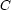, we want to speak (and possibly constrain) its truth value. Let's take an example from Chapter 5: the cardinality of a daughter set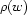is at most 1, and it is 1 iffis required by's valency, i.e if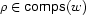. Let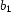stand for the truth value ofand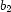stand for the truth value of: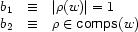The well-formedness condition mentioned above is expressed simply by.

For the purpose of this section, we will writeto represent a reified constraint, where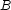is a FD variable representing the truth value of constraint: 0 means false, 1 means true. The operational semantics are as follows:

• ifis entailed then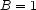is inferred

• ifis inconsistent then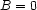is inferred

• ifis entailed, thenis imposed

• ifis entailed, then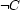is imposed

For example, here is how reified constraints can express that exactly one of,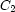, andmust hold: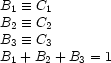Similarly, here is how to express:The astute reader may wonder why do we need a new concept? can't we express reified constraints in terms of disjunctive propagators?''. Indeed,can also be written:

or B=1 C [] B=0 ~C end

where somehow ~C is intended to represent the negation of C. What makes reified constraints attractive is that they are much more efficient. A disjunctive propagator needs to create 2 nested spaces, but a reified constraint doesn't need any.

In the libraries of the Mozart system, many constraints are also available as reified constraints (but not all). For example:

{FS.reified.include I S B}

B represents the truth value of {FS.include I S}. If B=0, the reified constraint reduces to {FS.exclude I S}.

Denys Duchier
Version 1.2.0 (20010221)